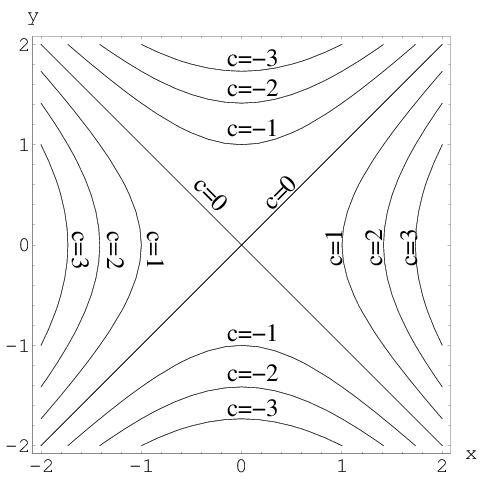# Math Insight

### Image: Level curves of a hyperbolic paraboloidLevel curves of $f(x,y)=x^2-y^2=c$ are hyperbolas.

Image file: level_curves_hyperbolic_paraboloid.png